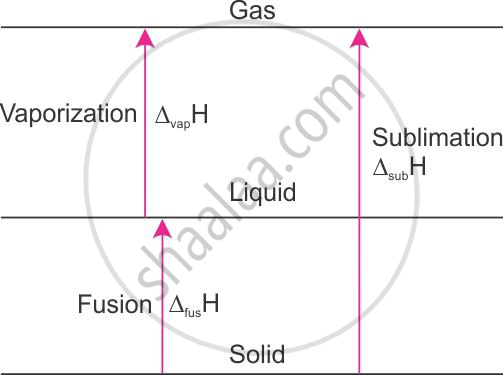HSC Science (General) 12th Board ExamMaharashtra State Board
Share

How is Enthalpy of Sublimation Related to Enthalpy of Fusion and Enthalpy of Vaporization - HSC Science (General) 12th Board Exam - Chemistry

ConceptChemical Thermodynamics and Energetic Internal Energy and Enthalpy

Question

How is enthalpy of sublimation related to enthalpy of fusion and enthalpy of vaporization?

Solution

Relation of enthalpy of sublimation with enthalpy of fusion and enthalpy of vaporisation:The enthalpy of sublimation of ice at 00C and 1 atm pressure is 51.08 kJ mol−1.H2O(s) → H2O(g), ΔH = 51.08 kJ mol−1 at 00C.

When solid is converted to vapour, either in one step or two steps, the solid first gets converted to the liquid state and then to the vapour state. The enthalpy change remains the same. This is because enthalpy is a state function.

For example,
H2O(s) → H2O(l), Δfus H = +6.01 kJ mol−1 at 0°C
H2O(l) → H2O(g), Δvap H = +45.07 kJ mol−1 at 0°C

_____________________________________

H2O(s) → H2O(g), Δ H = 51.08 kJ mol−1 at 0°C

Therefore, it follows that
Δsub H = Δfus H + Δvap H

Is there an error in this question or solution?

APPEARS IN

2015-2016 (March) (with solutions)
Question 1.6 | 2.00 marks
Solution How is Enthalpy of Sublimation Related to Enthalpy of Fusion and Enthalpy of Vaporization Concept: Chemical Thermodynamics and Energetic - Internal Energy and Enthalpy.
S